﻿ 移动端考研产品定价模型研究及应用 The Study and Applications of Mobile Terminal Postgraduate Product Pricing Model

Vol.07 No.05(2018), Article ID:25128,10 pages
10.12677/AAM.2018.75075

The Study and Applications of Mobile Terminal Postgraduate Product Pricing Model

Xiaoxiao Shen

Engineering College, Nanjing Agricultural University, Nanjing Jiangsu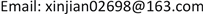Received: May 3rd, 2018; accepted: May 22nd, 2018; published: May 29th, 2018ABSTRACT

We have studied the pricing model of the mobile terminal product in this paper. First of all, we analyze the network externalities of the network information products; consumers expect effect and the positive feedback effect. After that, we construct the demand function of the mobile terminal postgraduate product and the utility function obtained by the candidate, so as to construct the revenue function and cost function of the market share of the mobile terminal postgraduate product. Starting from the law of changes between market share and corporate profits, we establish a pricing model for factor introduction. Finally, using the solution model, the marginal revenue curve and the marginal cost curve of the market share of the company’s mobile terminal product are obtained, and the parameter range is discussed, and the change of the curve is obtained. Based on the principle of market share first and profit share first, the two-phase pricing plan was obtained. And in this paper, the objective model’s advantages and disadvantages of the entire model are evaluated, as well as its application in other fields, which improves the practicality and practicality of the model.

Keywords:Mobile Terminal Examination Product, Period Factor, Pricing Model, Market Share, Profit Share1. 引言

2018年的全国硕士研究生考试共有238万人报名参加，比去年增加了37万名考生，增加18.4%，新一轮的考研热潮即将到来，而考研教学与培训的市场也发生了巨大的变化。移动互联网的到来，使得许多考研教学活动转移到了手机等移动互联网平台。现在的线上学习市场中，纷纷涌现了依托于移动互联网的产品，如教学app、手机题库、单词本、错题本或依托于现有移动端视频平台的直播课程等。公司如何抓住机会，迅速扩大市场占有率或利润占有率，对自己的移动端产品进行合理定价就显得十分重要。本文帮一家考研机构对其一款移动端产品进行全国定价。该产品的课程是由顶级名师讲授的，并配有配套的教材和在线答疑服务，预计每年的固定成本是300万元(不计市场推广费用)，分别以市场占有率优先和利润占有率优先为原则，为公司制定两套方案。

2. 问题分析

3. 模型的建立与求解

3.1. 模型假设

1) 企业的移动端产品可以即时获得来满足市场需求；

2) 移动端产品的边际成本为年固定成本的0.05%。

3.2. 符号说明

$P$ ：移动端考研产品的价格；

$\lambda$ ：消费者的支付意愿；

${Q}_{1}$ ：整个移动端考研产品产业的销量；

${Q}_{2}$ ：该公司的移动端考研产品的销量；

${C}_{1}$ ：该公司的生产成本；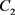：该公司的销售成本；

${t}_{i}$ ：公司所处的产业发展的第i个时期；

$e$ ：该公司移动端考研产品的市场占有率。

3.3. 基本概念分析

1) 正的网络外部性：网络信息产品正的网络外部性是指消费者消费信息产品所获得的效用随着购买这种信息产品的其他消费者数量的增加而不断增加，当网络信息产品的市场规模达到一定临界值时，需求方的规模经济和供给方的规模经济会产生良性互动，从而引发网络经济中的正反馈效应  。

2) 消费者预期效应和正反馈效应：正的网络外部性带来了消费者预期效应和正反馈效应。消费者对未来网络优势的预期即消费者预期效应对网络竞争优势的确立具有重要作用。在网络经济的一些领域中，供给方价格的下降会使需求增加，而需求的增加往往会创造更高的效率和报酬，从而导致供给方价格进一步下降，进而创造出更多的需求  。当市场由正反馈机制驱动时，其效率与其产业的整体规模成正比，由此发展出更大的网络，得以补偿高昂的初期固定成本，进而增加效率并减少平均成本，即一种产品的新的消费者可以给其他消费者带来正的外部收益，实现了需求方面的规模经济。

3.4. 引入时期因素的定价模型

3.4.1. 网络信息产品需求函数构建

$P=\alpha -\beta Q+\xi {Q}^{2}$ (1.1)

$\beta$ 代表消费者的实际边际消费倾向；

$\xi$ 代表消费者的预期边际消费倾向。

$\frac{\text{d}P}{\text{d}Q}=-\beta +2\xi Q$ (1.2)

$当Q>\beta /\left(2\xi \right)时，\frac{\text{d}P}{\text{d}Q}>0,$ 曲线斜率为正，向右上方倾斜；

$当Q=\beta /\left(2\xi \right)时，\frac{\text{d}P}{\text{d}Q}=0,即点\left(\frac{\beta }{2\xi },p\right)$ 为曲线的极小值点。

3.4.2. 网络信息产品消费者效用函数构建

${u}_{i}={b}_{i}+{a}_{i}{n}^{e}>0$

$U\left(q\right)=\theta q+\lambda {Q}^{e}q$ ( $\theta$ 为常数：给定的消费类型)

$U\left(\theta \right)=\theta q,q<{q}_{1},\lambda =0;$

$q\ge {q}^{*}$ 时，由于网络效应引发正反馈，越来越多的考生被吸引到移动端考研产品的购买中，使得移动端考研产品的购买规模扩大，考生所获得的总效用也不断扩大，因此，考生的支付意愿也随之扩大。我们将此段时期定义为 ${t}_{2}$ 时期，此时随着移动端考研产品购买规模达到临界点， $\lambda$ 不断变大。 ( $\left(\lambda \to 1,\lambda \in \left(0,1\right]\right)$ )，则有： $U\left(q\right)=\theta q+\lambda \left({B}_{O}+{Q}^{x}\right)q,\text{}{q}_{1}\le q<{q}_{2},\lambda \in \left(0,1\right]$ ；因此，可得到移动端考研产品的考生效用函数：

$U\left(q\right)=\left\{\begin{array}{l}\text{}\theta q\text{}q<{q}_{1},\lambda =0\\ \theta q+\lambda \left({B}_{0}+{Q}^{x}\right)q\text{}{q}_{1}\le q<{q}_{2},\lambda \in \left(0,1\right]\end{array}$ (1.3)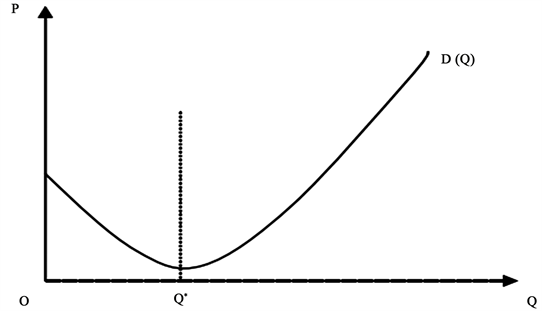Figure 1. Network information product demand curve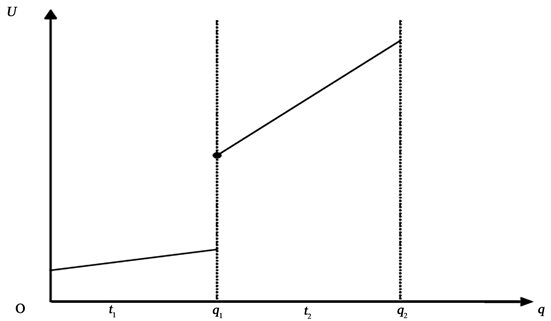Figure 2. Consumer utility curve

3.4.3. 构建移动端考研产品市场占有率的收益函数。

$e={Q}_{{}_{1}}/{Q}_{{}_{2}}$ (1.4)

$P=\alpha -\beta {Q}_{{}_{2}}+\xi {Q}_{{}_{2}}{}^{2}$ (1.5)

$R=e*{Q}_{{}_{1}}*\left(\alpha -\beta {Q}_{{}_{2}}+\xi {Q}_{2}^{2}\right)=\xi {Q}_{1}^{3}{e}^{3}-\beta {Q}_{1}^{2}{e}^{2}+\alpha {Q}_{{}_{1}}e$ (1.6)

$MR=\frac{\text{d}R}{\text{d}e}=3\xi {Q}_{1}^{3}{e}^{2}-2\beta {Q}_{1}^{2}e+\alpha {Q}_{{}_{1}}$ (1.7)

3.4.4. 构建该公司移动端考研产品市场占有率的成本函数。

$C={C}_{1}+{C}_{2}=3000000+1500{Q}_{{}_{2}}e+Ke=3000000+1500{e}^{2}{Q}_{{}_{1}}+Ke$ (1.8)

$MC=\frac{\text{d}C}{\text{d}e}=3000e{Q}_{{}_{1}}+K$ (1.9)

3.4.5. 模型的求解

$MR=MC$ 时，此时有最佳市场占有率。即 $3\xi {Q}_{1}^{3}{e}^{2}-2\beta {Q}_{1}^{2}e+\alpha {Q}_{{}_{1}}=3000e{Q}_{{}_{1}}+K$ 。模型中的三个参数α、β、 $\xi$ 均为大于零的自然数，其中代表消费者支付意愿的参数α (量纲：元)在短期内是恒定不变的，这里不妨假定α = 200元；下面讨论消费者最大消费量一定(这里不妨假定 ${Q}_{{}_{1}}=150000$ 个)、市场占有率一定(e = 20%)下，代表消费者实际边际消费倾向的参数β (量纲：元/个)与代表消费者预期边际消费倾向ξ (量纲：元/个)之间的关系发生变化时，由

$MR=\frac{\text{d}R}{\text{d}e}=3\xi {Q}_{1}^{3}{e}^{2}-2\beta {Q}_{1}^{2}e+\alpha {Q}_{{}_{1}}$

$\frac{\text{d}MR}{\text{d}e}=6\xi {Q}_{1}^{3}e-2\beta {Q}_{1}^{2}e=0,即3\xi {Q}_{{}_{1}}e-\beta =0$

$e>\frac{\beta }{3\xi {Q}_{{}_{1}}}时，\frac{\text{d}MR}{\text{d}e}>0$ ，曲线斜率为正，向右上方倾斜；

$e=\frac{\beta }{3\xi {Q}_{{}_{1}}}时，\frac{\text{d}MR}{\text{d}e}=0,即点\left(\frac{\beta }{3\xi {Q}_{{}_{1}}},P\right)$ 为曲线的极小值点。

1) $\beta <90000\xi$ 时，因为 $\beta ,\xi \in \left(0,1\right),$ 不妨选取 $\xi =\frac{1}{90000}$ ，此时 $\beta <90000\xi ⇒\beta <1$ ，而 $\forall \beta \in \left(0,1\right)$ ，当令 $\beta$ 分别取0.2, 0.4, 0.6, 0.8时，则仿真结果如下：

2) $\beta \ge 90000\xi$ 时因为 $\beta ,\xi \in \left(0,1\right),$ ，不妨选取 $\xi =\frac{1}{90000}$ 此时 $\beta \ge 90000\xi ⇒\beta \ge 0.1,$$\forall \beta \in \left(0,1\right)$ ，当令 $\beta$ 分别取0.2, 0.4, 0.6, 0.8时，则仿真结果如下：

4. 结果分析

4.1. 以市场占有率为优先原则的定价方案

${t}_{1}$ 时段，由于尚未达到临界点，网络效应很弱，消费者无法预期购买该种产品是一个正确的选择，消费者根据适应性预期无法预期它是标准。由 $MR=MC$ ，该时段对应于市场占有率的边际收益曲线与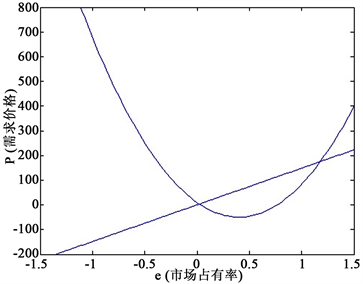Figure 3. Product demand curve at $\beta <90000\xi$ and β = 0.2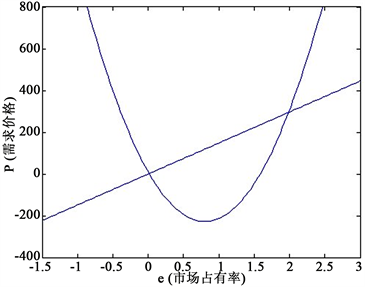Figure 4. Product demand curve at $\beta <90000\xi$ and β = 0.4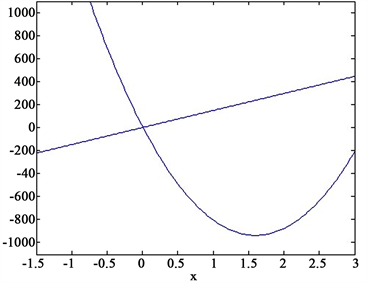Figure 5. Product demand curve at $\beta <90000\xi$ and β = 0.6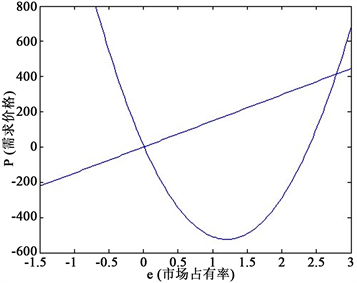Figure 6. Product demand curve at $\beta <90000\xi$ and β = 0.8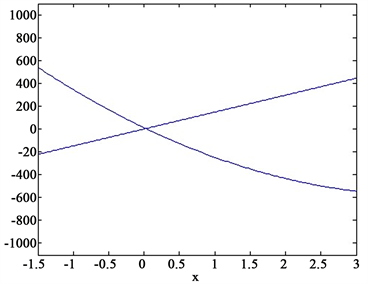Figure 7. Product demand curve at $\beta \ge 90000\xi$ and β = 0.2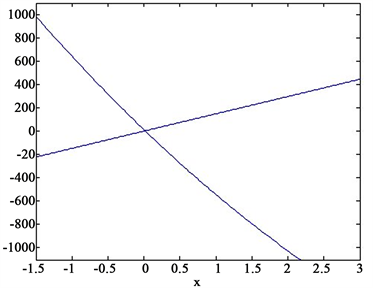Figure 8. Product demand curve at $\beta \ge 90000\xi$ and β = 0.4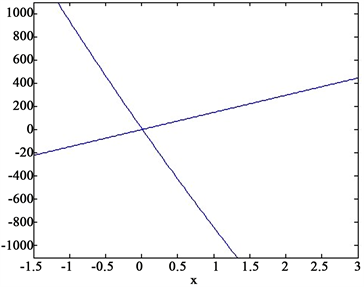Figure 9. Product demand curve at $\beta \ge 90000\xi$ and β = 0.6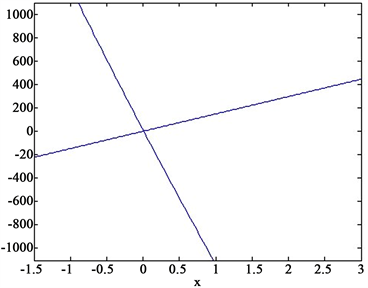Figure 10. Product demand curve at $\beta \ge 90000\xi$ and β = 0.8

4.2. 以利润率为优先原则的定价方案

${t}_{2}$ 时段，随着网络规模突破临界点，网络效应不断增强，引发正反馈，越来越多的消费者被吸引到网络中，形成良性循环。由 $MR=MC$ ，该时段对应于市场占有率的边际收益曲线与边际成本曲线的第二个交点。可以得到，在这一时期，公司的目标除了要不断扩大网络规模外，另一个重要目标应为强调锁定，增加消费者的总转移成本，将其锁定到本产品系列  。因此，对应的定价方案是隐形的定较高价格，来补偿 ${t}_{1}$ 时段。主要形式是大力销售高端版本，不断付费升级。运用捆绑定价，将消费者效用变化分布均匀化，从而导致消费者需求曲线在效用均值处更加具有弹性，造成锁定效果，增加消费者总的转移成本  。总之，在 ${t}_{2}$ 时段，公司应采用的定价方案是隐形的定高价，用 ${t}_{2}$ 时段的较高价补偿 ${t}_{1}$ 时段的较低价，实现利润最大化。

5. 模型的评价与推广

5.1. 模型的评价

5.2. 模型的推广

The Study and Applications of Mobile Terminal Postgraduate Product Pricing Model[J]. 应用数学进展, 2018, 07(05): 632-641. https://doi.org/10.12677/AAM.2018.75075

1. 1. 朱珠. 比特产品的需求与供给研究[D]: [博士学位论文]. 北京: 北京邮电大学, 2013.

2. 2. 刘小丽. 基于促销影响的定价模型研究[D]: [硕士学位论文]. 沈阳: 东北大学, 2010.

3. 3. 罗韬. 论产业成长期企业市场占有率与利润率的战略权衡[D]: [硕士学位论文]. 湘潭: 湘潭大学, 2007.

4. 4. 缪谦. 网络信息产品定价研究[D]: [硕士学位论文]. 成都: 西南财经大学, 2008.

5. 5. 马秋楠. 比特经济学研究综述[D]: [硕士学位论文]. 北京: 北京邮电大学, 2009.

6. 6. Hadjimatheou, G. (1987) Consumer Economics after Keynes: Theory and Evidence of the Consumption Function.